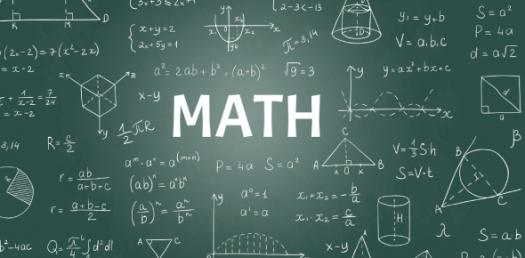# Integrated Math 1 Pre-test

40 Questions | Attempts: 1352SettingsThis pre-test is to help me get an understanding of the math skills you have acquired prior to this course. Please attempt all problems that you can. There is not a passing grade for this test, so even if you think you are wrong - just do your best to show me what you do or do not know!

• 1.
The bill for your dinner is \$45.78.  If you want to include a 15% tip, what will the total for dinner and tip be?
• A.

\$52.64

• B.

\$52.65

• C.

\$6.86

• D.

\$6.87

• 2.
In nine years Sarah will be 15 years old.  How old is Sarah now?
• 3.
Add the following: 2/3 and 4/5.
• A.

22/15

• B.

6/8

• C.

29/15

• D.

6/15

• 4.
Which of the following is a perfect square?
• A.

6

• B.

12

• C.

25

• D.

32

• 5.
There are 2.54 cm in one inch. How many cm are in six yards?
• 6.
What is the absolute value of 3-7? [ 3 - 7 ] =
• 7.
Put these numbers in order from smallest to largest: 0.5, 0.05, 0.15, 1.0
• 8.
23/4 + 6 * 3 = Remember to use the order of operations. (  / means divided by)
• 9.
Evaluate 4x - 6 when x = 5.
• 10.
A rectangle has a length of twelve inches and a width of four inches.  What is the perimeter?
• 11.
What is the slope of the line with equation y = 3x - 10?
• A.

-10

• B.

10

• C.

3

• D.

-3

• 12.
There are 54 students going on a field trip.  If each van can carry six students, how many vans will be necessary to carry all students?
• 13.
Change 6 2/7 (six and two sevenths) to an improper fraction instead of a mixed number.
• 14.
4 + (-7) + 2 + (-5) = ?
• 15.
Use the Pythagorean Theorem to find the hypotenuse of a right triangle with legs that measure 3 and 4.
• A.

5

• B.

25

• C.

7

• D.

49

• 16.
What is the product of 49 written as prime factors?
• A.

49 * 1

• B.

7*7

• C.

1 * (48 + 1)

• 17.
What is the greatest common factor of 12 & 28?
• 18.
Round 40.5656 to the nearest tenth.
• 19.
Change 5/1000 to a decimal.
• 20.
Which of the following is the correct volume for a cube which has sides that mesure 3 in.?
• A.

6 cubic inches

• B.

9 cubic inches

• C.

15 cubic inches

• D.

27 cubic inches

• 21.
Evaluate (- x)2 when x = 3.
• 22.
1/3 = _____ % Round to the nearest whole percent.
• 23.
What percent of 20 = 8?
• 24.
If r = 5 and s = 8, what is the value of r2 - 2s?
• 25.
Write the decimal number which represents "two and sixteen thousandths".

## Related TopicsBack to top
×

Wait!
Here's an interesting quiz for you.